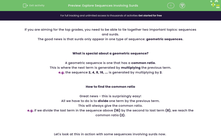# Explore Sequences Involving Surds

In this worksheet, students will find the common ratios in sequences involving surds, apply these to find missing terms and explore sequential relationships.Key stage:  KS 4

Year:  GCSE

GCSE Subjects:   Maths

GCSE Boards:   AQA, Eduqas, OCR, Pearson Edexcel,

Curriculum topic:   Algebra

Curriculum subtopic:   Sequences

Difficulty level:#### Worksheet Overview

If you are aiming for the top grades, you need to be able to tie together two important topics: sequences and surds.

The good news is that surds only appear in one type of sequence: geometric sequences.

What is special about a geometric sequence?

A geometric sequence is one that has a common ratio.

This is where the next term is generated by multiplying the previous term.

e.g. the sequence 2, 4, 8, 16, ... is generated by multiplying by 2.

How to find the common ratio

Great news - this is surprisingly easy!

All we have to do is to divide one term by the previous term.

This will always give the common ratio.

e.g. if we divide the last term in the sequence above (16) by the second to last term (8), we reach the common ratio (2).

Let's look at this in action with some sequences involving surds now.

e.g. Continue the sequence √3, 3, 3√3, 9, ...

Step 1: Find the common ratio.

We're going to pick the third and second terms for this, just because the numbers are easier to work with.

You can pick whichever ones you choose to find the common ratio.

3√3 ÷ 3 = √3

So my common ratio is √3.

Step 2: Continue the sequence.

Now we need to multiply the fourth term by √3 to get the fifth term:

9 × √3 = 9√3

Then I can multiply this fifth term by √3 to get the sixth term:

9√3 × √3 = 9 × 3 = 27

In this activity, you will find the common ratios in sequences involving surds and apply these to find missing terms and explore sequential relationships.

### What is EdPlace?

We're your National Curriculum aligned online education content provider helping each child succeed in English, maths and science from year 1 to GCSE. With an EdPlace account you’ll be able to track and measure progress, helping each child achieve their best. We build confidence and attainment by personalising each child’s learning at a level that suits them.

Get started